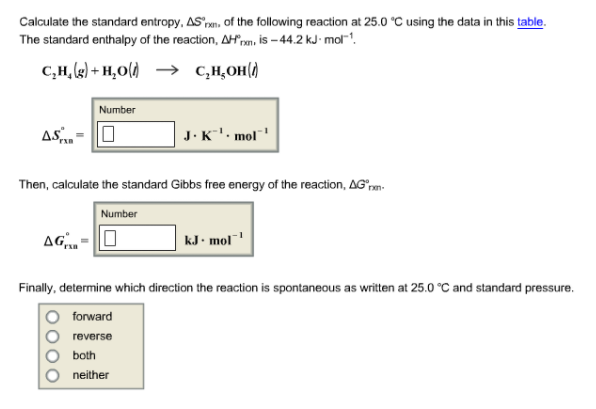# Problem: Calculate the standard entropy, ΔSorxn of the following reaction at 25.0 0C. The standard enthalpy of the reaction, ΔHorxn, is -44.2 kJ/mol.

###### FREE Expert Solution###### Problem Details

Calculate the standard entropy, ΔSorxn of the following reaction at 25.0 0C. The standard enthalpy of the reaction, ΔHorxn, is -44.2 kJ/mol.What scientific concept do you need to know in order to solve this problem?

Our tutors have indicated that to solve this problem you will need to apply the Gibbs Free Energy concept. You can view video lessons to learn Gibbs Free Energy. Or if you need more Gibbs Free Energy practice, you can also practice Gibbs Free Energy practice problems.

What is the difficulty of this problem?

Our tutors rated the difficulty ofCalculate the standard entropy, ΔSorxn of the following reac...as high difficulty.

How long does this problem take to solve?

Our expert Chemistry tutor, Dasha took 7 minutes and 57 seconds to solve this problem. You can follow their steps in the video explanation above.

What professor is this problem relevant for?

Based on our data, we think this problem is relevant for Professor Leslie's class at UCM.